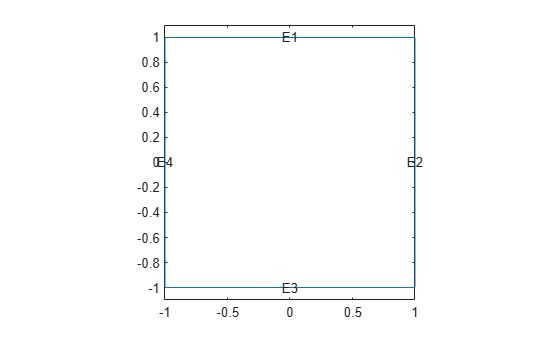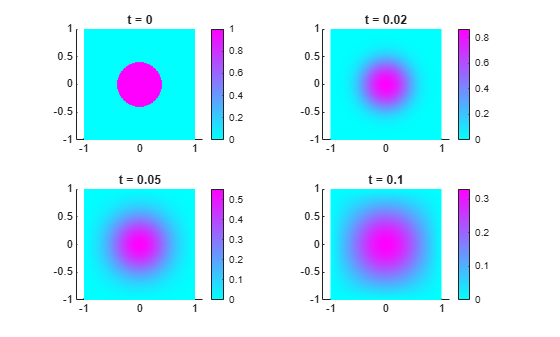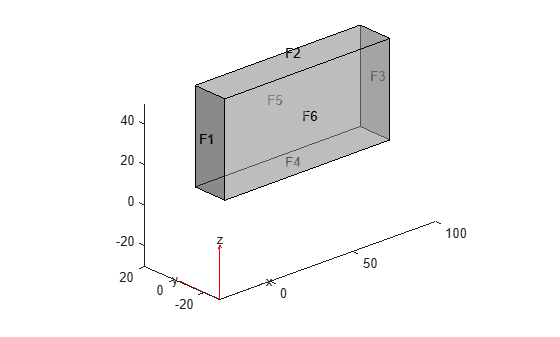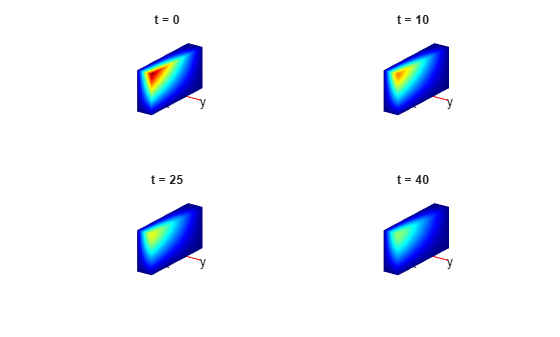# parabolic

(Not recommended) Solve parabolic PDE problem

`parabolic` is not recommended. Use `solvepde` instead.

## Syntax

``u = parabolic(u0,tlist,model,c,a,f,d)``
``u = parabolic(u0,tlist,b,p,e,t,c,a,f,d)``
``u = parabolic(u0,tlist,Kc,Fc,B,ud,M)``
``u = parabolic(___,rtol)``
``````u = parabolic(___,rtol,atol)``````
``u = parabolic(___,'Stats','off')``

## Description

Parabolic equation solver

Solves PDE problems of the type

`$d\frac{\partial u}{\partial t}-\nabla \cdot \left(c\nabla u\right)+au=f$`

on a 2-D or 3-D region Ω, or the system PDE problem

`$d\frac{\partial u}{\partial t}-\nabla \cdot \left(c\otimes \nabla u\right)+au=f$`

The variables c, a, f, and d can depend on position, time, and the solution u and its gradient.

example

````u = parabolic(u0,tlist,model,c,a,f,d)` produces the solution to the FEM formulation of the scalar PDE problem$d\frac{\partial u}{\partial t}-\nabla \cdot \left(c\nabla u\right)+au=f$on a 2-D or 3-D region Ω, or the system PDE problem $d\frac{\partial u}{\partial t}-\nabla \cdot \left(c\otimes \nabla u\right)+au=f$with geometry, mesh, and boundary conditions specified in `model`, and with initial value `u0`. The variables c, a, f, and d in the equation correspond to the function coefficients `c`, `a`, `f`, and `d` respectively.```

example

````u = parabolic(u0,tlist,b,p,e,t,c,a,f,d)` solves the problem using boundary conditions `b` and finite element mesh specified in `[p,e,t]`.```

example

````u = parabolic(u0,tlist,Kc,Fc,B,ud,M)` solves the problem based on finite element matrices that encode the equation, mesh, and boundary conditions.```
````u = parabolic(___,rtol)` and ```u = parabolic(___,rtol,atol)```, for any of the previous input arguments, modify the solution process by passing to the ODE solver a relative tolerance `rtol`, and optionally an absolute tolerance `atol`.```
````u = parabolic(___,'Stats','off')`, for any of the previous input arguments, turns off the display of internal ODE solver statistics during the solution process.```

## Examples

collapse all

Solve the parabolic equation

`$\frac{\partial u}{\partial t}=\Delta u$`

on the square domain specified by `squareg`.

Create a PDE model and import the geometry.

```model = createpde; geometryFromEdges(model,@squareg); pdegplot(model,'EdgeLabels','on') ylim([-1.1,1.1]) axis equal```Set Dirichlet boundary conditions $u=0$ on all edges.

```applyBoundaryCondition(model,'dirichlet',... 'Edge',1:model.Geometry.NumEdges, ... 'u',0);```

Generate a relatively fine mesh.

`generateMesh(model,'Hmax',0.02,'GeometricOrder','linear');`

Set the initial condition to have $u\left(0\right)=1$ on the disk ${x}^{2}+{y}^{2}\le 0.{4}^{2}$ and $u\left(0\right)=0$ elsewhere.

```p = model.Mesh.Nodes; u0 = zeros(size(p,2),1); ix = find(sqrt(p(1,:).^2 + p(2,:).^2) <= 0.4); u0(ix) = ones(size(ix));```

Set solution times to be from 0 to 0.1 with step size 0.005.

`tlist = linspace(0,0.1,21);`

Create the PDE coefficients.

```c = 1; a = 0; f = 0; d = 1;```

Solve the PDE.

`u = parabolic(u0,tlist,model,c,a,f,d);`
```133 successful steps 0 failed attempts 268 function evaluations 1 partial derivatives 26 LU decompositions 267 solutions of linear systems ```

Plot the initial condition, the solution at the final time, and two intermediate solutions.

```figure subplot(2,2,1) pdeplot(model,'XYData',u(:,1)); axis equal title('t = 0') subplot(2,2,2) pdeplot(model,'XYData',u(:,5)) axis equal title('t = 0.02') subplot(2,2,3) pdeplot(model,'XYData',u(:,11)) axis equal title('t = 0.05') subplot(2,2,4) pdeplot(model,'XYData',u(:,end)) axis equal title('t = 0.1')```Solve the parabolic equation

`$\frac{\partial u}{\partial t}=\Delta u$`

on the square domain specified by `squareg`, using a geometry function to specify the geometry, a boundary function to specify the boundary conditions, and using `initmesh` to create the finite element mesh.

Specify the geometry as `@squareg` and plot the geometry.

```g = @squareg; pdegplot(g,'EdgeLabels','on') ylim([-1.1,1.1]) axis equal```Set Dirichlet boundary conditions $u=0$ on all edges. The `squareb1` function specifies these boundary conditions.

`b = @squareb1;`

Generate a relatively fine mesh.

`[p,e,t] = initmesh(g,'Hmax',0.02);`

Set the initial condition to have $u\left(0\right)=1$ on the disk ${x}^{2}+{y}^{2}\le 0.{4}^{2}$ and $u\left(0\right)=0$ elsewhere.

```u0 = zeros(size(p,2),1); ix = find(sqrt(p(1,:).^2 + p(2,:).^2) <= 0.4); u0(ix) = ones(size(ix));```

Set solution times to be from 0 to 0.1 with step size 0.005.

`tlist = linspace(0,0.1,21);`

Create the PDE coefficients.

```c = 1; a = 0; f = 0; d = 1;```

Solve the PDE.

`u = parabolic(u0,tlist,b,p,e,t,c,a,f,d);`
```147 successful steps 0 failed attempts 296 function evaluations 1 partial derivatives 28 LU decompositions 295 solutions of linear systems ```

Plot the initial condition, the solution at the final time, and two intermediate solutions.

```figure subplot(2,2,1) pdeplot(p,e,t,'XYData',u(:,1)); axis equal title('t = 0') subplot(2,2,2) pdeplot(p,e,t,'XYData',u(:,5)) axis equal title('t = 0.02') subplot(2,2,3) pdeplot(p,e,t,'XYData',u(:,11)) axis equal title('t = 0.05') subplot(2,2,4) pdeplot(p,e,t,'XYData',u(:,end)) axis equal title('t = 0.1')```Create finite element matrices that encode a parabolic problem, and solve the problem.

The problem is the evolution of temperature in a conducting block. The block is a rectangular slab.

```model = createpde(1); importGeometry(model,'Block.stl'); handl = pdegplot(model,'FaceLabels','on'); view(-42,24) handl(1).FaceAlpha = 0.5;```Faces 1, 4, and 6 of the slab are kept at 0 degrees. The other faces are insulated. Include the boundary condition on faces 1, 4, and 6. You do not need to include the boundary condition on the other faces because the default condition is insulated.

`applyBoundaryCondition(model,'dirichlet','Face',[1,4,6],'u',0);`

The initial temperature distribution in the block has the form

`${u}_{0}=1{0}^{-3}xyz.$`

```generateMesh(model); p = model.Mesh.Nodes; x = p(1,:); y = p(2,:); z = p(3,:); u0 = x.*y.*z*1e-3;```

The parabolic equation in toolbox syntax is

`$d\frac{\partial u}{\partial t}-\nabla \cdot \left(c\nabla u\right)+au=f.$`

Suppose the thermal conductivity of the block leads to a $c$ coefficient value of 1. The values of the other coefficients in this problem are $d=1$, $a=0$, and $f=0$.

```d = 1; c = 1; a = 0; f = 0;```

Create the finite element matrices that encode the problem.

```[Kc,Fc,B,ud] = assempde(model,c,a,f); [~,M,~] = assema(model,0,d,f);```

Solve the problem at time steps of 1 for times ranging from 0 to 40.

```tlist = linspace(0,40,41); u = parabolic(u0,tlist,Kc,Fc,B,ud,M);```
```35 successful steps 0 failed attempts 72 function evaluations 1 partial derivatives 11 LU decompositions 71 solutions of linear systems ```

Plot the solution on the outside of the block at times 0, 10, 25, and 40. Ensure that the coloring is the same for all plots.

```umin = min(min(u)); umax = max(max(u)); subplot(2,2,1) pdeplot3D(model,'ColorMapData',u(:,1)) colorbar off view(125,22) title 't = 0' caxis([umin umax]); subplot(2,2,2) pdeplot3D(model,'ColorMapData',u(:,11)) colorbar off view(125,22) title 't = 10' caxis([umin umax]); subplot(2,2,3) pdeplot3D(model,'ColorMapData',u(:,26)) colorbar off view(125,22) title 't = 25' caxis([umin umax]); subplot(2,2,4) pdeplot3D(model,'ColorMapData',u(:,41)) colorbar off view(125,22) title 't = 40' caxis([umin umax]);```## Input Arguments

collapse all

Initial condition, specified as a scalar, vector of nodal values, character vector, character array, string scalar, or string vector. The initial condition is the value of the solution `u` at the initial time, specified as a column vector of values at the nodes. The nodes are either `p` in the `[p,e,t]` data structure, or are `model.Mesh.Nodes`.

• If the initial condition is a constant scalar `v`, specify `u0` as `v`.

• If there are `Np` nodes in the mesh, and N equations in the system of PDEs, specify `u0` as a column vector of `Np`*N elements, where the first `Np` elements correspond to the first component of the solution `u`, the second `Np` elements correspond to the second component of the solution `u`, etc.

• Give a text expression of a function, such as ```'x.^2 + 5*cos(x.*y)'```. If you have a system of N > 1 equations, give a text array such as

```char('x.^2 + 5*cos(x.*y)',... 'tanh(x.*y)./(1+z.^2)')```

Example: `x.^2+5*cos(y.*x)`

Data Types: `double` | `char` | `string`
Complex Number Support: Yes

Solution times, specified as a real vector. The solver returns the solution to the PDE at the solution times.

Example: `0:0.2:4`

Data Types: `double`

PDE model, specified as a `PDEModel` object.

Example: `model = createpde`

PDE coefficient, specified as a scalar, matrix, character vector, character array, string scalar, string vector, or coefficient function. `c` represents the c coefficient in the scalar PDE

`$d\frac{\partial u}{\partial t}-\nabla \cdot \left(c\nabla u\right)+au=f$`

or in the system of PDEs

`$d\frac{\partial u}{\partial t}-\nabla \cdot \left(c\otimes \nabla u\right)+au=f$`

Example: `'cosh(x+y.^2)'`

Data Types: `double` | `char` | `string` | `function_handle`
Complex Number Support: Yes

PDE coefficient, specified as a scalar, matrix, character vector, character array, string scalar, string vector, or coefficient function. `a` represents the a coefficient in the scalar PDE

`$d\frac{\partial u}{\partial t}-\nabla \cdot \left(c\nabla u\right)+au=f$`

or in the system of PDEs

`$d\frac{\partial u}{\partial t}-\nabla \cdot \left(c\otimes \nabla u\right)+au=f$`

Example: `2*eye(3)`

Data Types: `double` | `char` | `string` | `function_handle`
Complex Number Support: Yes

PDE coefficient, specified as a scalar, matrix, character vector, character array, string scalar, string vector, or coefficient function. `f` represents the f coefficient in the scalar PDE

`$d\frac{\partial u}{\partial t}-\nabla \cdot \left(c\nabla u\right)+au=f$`

or in the system of PDEs

`$d\frac{\partial u}{\partial t}-\nabla \cdot \left(c\otimes \nabla u\right)+au=f$`

Example: `char('sin(x)';'cos(y)';'tan(z)')`

Data Types: `double` | `char` | `string` | `function_handle`
Complex Number Support: Yes

PDE coefficient, specified as a scalar, matrix, character vector, character array, string scalar, string vector, or coefficient function. `d` represents the d coefficient in the scalar PDE

`$d\frac{\partial u}{\partial t}-\nabla \cdot \left(c\nabla u\right)+au=f$`

or in the system of PDEs

`$d\frac{\partial u}{\partial t}-\nabla \cdot \left(c\otimes \nabla u\right)+au=f$`

Example: `2*eye(3)`

Data Types: `double` | `char` | `string` | `function_handle`
Complex Number Support: Yes

Boundary conditions, specified as a boundary matrix or boundary file. Pass a boundary file as a function handle or as a file name. A boundary matrix is generally an export from the PDE Modeler app.

Example: `b = 'circleb1'`, `b = "circleb1"`, or ```b = @circleb1```

Data Types: `double` | `char` | `string` | `function_handle`

Mesh points, specified as a 2-by-`Np` matrix of points, where `Np` is the number of points in the mesh. For a description of the (`p`,`e`,`t`) matrices, see Mesh Data as [p,e,t] Triples.

Typically, you use the `p`, `e`, and `t` data exported from the PDE Modeler app, or generated by `initmesh` or `refinemesh`.

Example: `[p,e,t] = initmesh(gd)`

Data Types: `double`

Mesh edges, specified as a `7`-by-`Ne` matrix of edges, where `Ne` is the number of edges in the mesh. For a description of the (`p`,`e`,`t`) matrices, see Mesh Data as [p,e,t] Triples.

Typically, you use the `p`, `e`, and `t` data exported from the PDE Modeler app, or generated by `initmesh` or `refinemesh`.

Example: `[p,e,t] = initmesh(gd)`

Data Types: `double`

Mesh triangles, specified as a `4`-by-`Nt` matrix of triangles, where `Nt` is the number of triangles in the mesh. For a description of the (`p`,`e`,`t`) matrices, see Mesh Data as [p,e,t] Triples.

Typically, you use the `p`, `e`, and `t` data exported from the PDE Modeler app, or generated by `initmesh` or `refinemesh`.

Example: `[p,e,t] = initmesh(gd)`

Data Types: `double`

Stiffness matrix, specified as a sparse matrix or as a full matrix. See Elliptic Equations. Typically, `Kc` is the output of `assempde`.

Load vector, specified as a vector. See Elliptic Equations. Typically, `Fc` is the output of `assempde`.

Dirichlet nullspace, returned as a sparse matrix. See Algorithms. Typically, `B` is the output of `assempde`.

Dirichlet vector, returned as a vector. See Algorithms. Typically, `ud` is the output of `assempde`.

Mass matrix. specified as a sparse matrix or a full matrix. See Elliptic Equations.

To obtain the input matrices for `pdeeig`, `hyperbolic` or `parabolic`, run both `assema` and `assempde`:

```[Kc,Fc,B,ud] = assempde(model,c,a,f); [~,M,~] = assema(model,0,d,f);```

Note

Create the `M` matrix using `assema` with `d`, not `a`, as the argument before `f`.

Data Types: `double`
Complex Number Support: Yes

Relative tolerance for ODE solver, specified as a positive real.

Example: `2e-4`

Data Types: `double`

Absolute tolerance for ODE solver, specified as a positive real.

Example: `2e-7`

Data Types: `double`

## Output Arguments

collapse all

PDE solution, returned as a matrix. The matrix is `Np`*N-by-`T`, where `Np` is the number of nodes in the mesh, N is the number of equations in the PDE (N = 1 for a scalar PDE), and `T` is the number of solution times, meaning the length of `tlist`. The solution matrix has the following structure.

• The first `Np` elements of each column in `u` represent the solution of equation 1, then next `Np` elements represent the solution of equation 2, etc. The solution `u` is the value at the corresponding node in the mesh.

• Column `i` of `u` represents the solution at time `tlist``(i)`.

To obtain the solution at an arbitrary point in the geometry, use `pdeInterpolant`.

To plot the solution, use `pdeplot` for 2-D geometry, or see 3-D Solution and Gradient Plots with MATLAB Functions.

## Algorithms

collapse all

### Reducing Parabolic Equations to Elliptic Equations

`parabolic` internally calls `assema`, `assemb`, and `assempde` to create finite element matrices corresponding to the problem. It calls `ode15s` to solve the resulting system of ordinary differential equations.

Partial Differential Equation Toolbox™ solves equations of the form

`$m\frac{{\partial }^{2}u}{\partial {t}^{2}}+d\frac{\partial u}{\partial t}-\nabla ·\left(c\nabla u\right)+au=f$`

When the m coefficient is 0, but d is not, the documentation refers to the equation as parabolic, whether or not it is mathematically in parabolic form.

A parabolic problem is to solve the equation

with the initial condition

u(x,0) = u0(x) for x∊Ω

where x represents a 2-D or 3-D point and there are boundary conditions of the same kind as for the elliptic equation on ∂Ω.

`$\rho C\frac{\partial u}{\partial t}-\nabla \text{\hspace{0.17em}}·\text{\hspace{0.17em}}\left(k\nabla u\right)+h\left(u-{u}_{\infty }\right)=f$`

in the presence of distributed heat loss to the surroundings. ρ is the density, C is the thermal capacity, k is the thermal conductivity, h is the film coefficient, u is the ambient temperature, and f is the heat source.

For time-independent coefficients, the steady-state solution of the equation is the solution to the standard elliptic equation

–∇ · (cu) + au = f.

Assuming a mesh on Ω and t ≥ 0, expand the solution to the PDE (as a function of x) in the Finite Element Method basis:

`$u\left(x,t\right)=\sum _{i}{U}_{i}\left(t\right){\varphi }_{i}\left(x\right)$`

Plugging the expansion into the PDE, multiplying with a test function ϕj, integrating over Ω, and applying Green's formula and the boundary conditions yield

`$\begin{array}{l}\sum _{i}\underset{\Omega }{\int }d{\varphi }_{j}{\varphi }_{i}\text{\hspace{0.17em}}\frac{d{U}_{i}\left(t\right)}{dt}\text{\hspace{0.17em}}dx+\sum _{i}\left(\underset{\Omega }{\int }\left(\nabla {\varphi }_{j}\cdot \left(c\nabla {\varphi }_{i}\right)+a{\varphi }_{j}{\varphi }_{i}\right)\text{\hspace{0.17em}}dx+\underset{\partial \Omega }{\int }q{\varphi }_{j}{\varphi }_{i}\text{\hspace{0.17em}}ds\right){U}_{i}\left(t\right)\\ \text{ }\text{ }\text{ }\text{ }=\underset{\Omega }{\int }f{\varphi }_{j}\text{\hspace{0.17em}}dx+\underset{\partial \Omega }{\int }g{\varphi }_{j}\text{\hspace{0.17em}}ds\text{ }\forall j\end{array}$`

In matrix notation, we have to solve the linear, large and sparse ODE system

`$M\frac{dU}{dt}+KU=F$`

This method is traditionally called method of lines semidiscretization.

Solving the ODE with the initial value

Ui(0) = u0(xi)

yields the solution to the PDE at each node xi and time t. Note that K and F are the stiffness matrix and the right-hand side of the elliptic problem

–∇ · (cu) + au = f in Ω

with the original boundary conditions, while M is just the mass matrix of the problem

–∇ · (0∇u) + du = 0 in Ω.

When the Dirichlet conditions are time dependent, F contains contributions from time derivatives of h and r. These derivatives are evaluated by finite differences of the user-specified data.

The ODE system is ill conditioned. Explicit time integrators are forced by stability requirements to very short time steps while implicit solvers can be expensive since they solve an elliptic problem at every time step. The numerical integration of the ODE system is performed by the MATLAB® ODE Suite functions, which are efficient for this class of problems. The time step is controlled to satisfy a tolerance on the error, and factorizations of coefficient matrices are performed only when necessary. When coefficients are time dependent, the necessity of reevaluating and refactorizing the matrices each time step may still make the solution time consuming, although `parabolic` reevaluates only that which varies with time. In certain cases a time-dependent Dirichlet matrix h(t) may cause the error control to fail, even if the problem is mathematically sound and the solution u(t) is smooth. This can happen because the ODE integrator looks only at the reduced solution v with u = Bv + ud. As h changes, the pivoting scheme employed for numerical stability may change the elimination order from one step to the next. This means that B, v, and ud all change discontinuously, although u itself does not.

## Version History

Introduced before R2006a